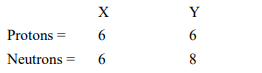# Composition of the nuclei of two atomic

Question.
Composition of the nuclei of two atomic species X and Y are given as underGive the mass numbers of X and Y. What is the relation between the two species?

Solution:

Mass number of X = Number of protons + Number of neutrons = 6 + 6 = 12
Mass number of Y = Number of protons + Number of neutrons = 6 + 8 = 14
These two atomic species X and Y have the same atomic number, but different mass numbers.
Hence, they are isotopes.